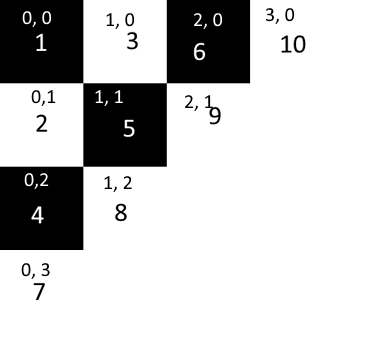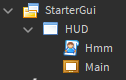# How to automatically do a checkerboard pattern

I am wanting to create a checkboard pattern that appears on screen procedurally, so like

frame 1 would appear first, then 2, then 3, etc. until the whole screen was covered

I’m trying to just figure out the right math for it, and so at just 2 for the amount, which creates 3 frames, so frames 1, 2, 3 should be there

``````local Amount = 2

local Sizing = HUD.AbsoluteSize.X / 12

for g = 1, Amount do
for i = 1, g do
local NewFrame = Instance.new("Frame")
NewFrame.BackgroundColor3 = Color3.fromRGB(0, 0, 0)
NewFrame.BorderSizePixel = 0
NewFrame.Size = UDim2.fromOffset(Sizing, Sizing)

NewFrame.Position = UDim2.fromOffset((i - 1) * Sizing, (i - 1) * Sizing)

NewFrame.Parent = HUD.Frame

end
end
``````

It creates 2 frames at 0, 0, and then the 3rd at 159, 159.

``````local whiteSquare = true

for I=1, N do
for i=1,n do
local newFrame = Instance.new("Frame")
newFrame.BackgroundColor3 = (whiteSquare and Color3.fromRGB(255,255,255)) or Color3.fromRGB(255,255,255)
newFrame.BorderSizePixel = 0
newFrame.Size = UDim2.fromOffset(TheSize, TheSize)
newFrame.Position = UDim2.fromOffset((idkwhatthisis) * TheSize, (idkwhatthisis) * TheSize)
newFrame.Parent = HUD.Frame
whiteSquare = not whiteSquare
end
end
``````
1 Like

What is N and n??? You haven’t really answered the question. I am asking about positional stuff, not making the tiles colored. I know how to do that

1 Like

N and n as in number (you defined it as Amount and g in your code)

Also apologies, to make the code completer:

``````local whiteSquare = true
local targetFrame = --shrug

for x=0, targetFrame.X.Offset, singleFrameSizeWidth do
for y=0, targetFrame.Y.Offset, singleFrameSizeLength do
local newFrame = Instance.new("Frame")
newFrame.BackgroundColor3 = (whiteSquare and Color3.fromRGB(255,255,255)) or Color3.fromRGB(255,255,255)
newFrame.BorderSizePixel = 0
newFrame.Size = UDim2.fromOffset(singleFrameSizeWidth, singleFrameSizeLength)
newFrame.Position = UDim2.fromOffset(x, y)
newFrame.Parent = targetFrame
whiteSquare = not whiteSquare
end
end
``````
1 Like

Close, but I want it to angle, like in my picture in the question.

1 Like

Hopefully this code helps?

``````for y=0, amount -1 do
local color = Color3.new(1, 1, 1)

if y % 2 == 0 then
color = Color3.new(0, 0, 0)

end

for x=0, y do
local frame = Instance.new("Frame")
frame.Size = UDim2.fromOffset(size, size)
frame.Position = UDim2.fromOffset(x * size, (x - y) * size)
frame.BackgroundColor3 = color
frame.Parent = script.Parent

wait(0.5)

end
end
``````
1 Like

Just adds frames to top of screen, doesn’t tile them

1 Like

My bad! I messed up the order of the subtration!

``````for y=0, amount -1 do
local color = Color3.new(1, 1, 1)

if y % 2 == 0 then
color = Color3.new(0, 0, 0)

end

for x=0, y do
local frame = Instance.new("Frame")
frame.Size = UDim2.fromOffset(size, size)
frame.Position = UDim2.fromOffset(x * size, (y - x) * size)
frame.BackgroundColor3 = color
frame.Parent = script.Parent

wait(0.5)

end
end
``````

May I ask how you were able to pertain this mathematical knowledge

if you want it to be like a raster this is very easy you just use ui grid layout

Yeah sure! So basically, the pattern here is:Now you start out on the side all the way down, and then you move one to the right, and one to the up every time. This is why when x adds one, you add x to the right, and subtract x up from the last position.

Could elaborate more if it didn’t make sense.

Wouldn’t it then only cast half the checkerboard pattern?

oh yea, but what you can do is you can limit the x to the max amount that fits I think?

Could you elaborate, please? I thought of just multiplying amount and checking if the x/y is on overflow of amount.

Ok I unfortunately didn’t get anything to work, I’m sorry. My idea was that after the half has been completed, you could run a special one filling in the gaps.

You’ll need two for loops (which I see you’ve got), one to handle rows the other to handle columns.

He wants it diagonally, which you could read from the previous post.
I don’t see how your post would solve his diagonal filling issue. (Vocrus couldn’t find it out hwo to complete the other half either)

Instead of an algorithm to do it you could just write it all out manually.

I finally got it!

``````local amount_x = 10
local amount_y = 7
local size = 25
local black = true

function CreateFrame(x, y)
local frame = Instance.new("Frame")
frame.Size = UDim2.fromOffset(size, size)
frame.Position = UDim2.fromOffset(x * size, y * size)
frame.BackgroundColor3 = (black and Color3.new(0, 0, 0)) or Color3.new(1, 1, 1)
frame.Parent = script.Parent

end

function FillLine(startX, startY, len)
for dir=0, len - 1 do
local x = startX + dir
local y = startY - dir
print(x, y)
CreateFrame(x, y)
wait(0.1)

end
black = not black
end

for y=0, amount_y -1 do
FillLine(0, y, math.min(y + 1, amount_x))
end

for x=1, amount_x - 1 do
local length = math.min(amount_y, amount_x - x )
FillLine(x, amount_y - 1, length)

end

``````

I got something that I would consider similar to what OP wanted, but I used a different approach and I feel like my answer is so close, I’m just missing something. I used a kinda ‘stitched’ together method for this lol so beware.

GIF of mine running:

https://gyazo.com/79fcf6459a4d2f34d7989fed94897ed1

The code that I used:

``````local TweenService = game:GetService("TweenService")
local HUD = script.Parent

local Amount = 12
local Sizing = HUD.AbsoluteSize.X / Amount

local PreviousTile = nil
local Count = 0

task.wait(3) -- so I can watch the effect

local function ni(a,b,c,d)
local i=Instance.new(a)
if c then
i.Name=c
end
if d then
for p,v in pairs(d) do
i[p]=v
end
end
if b then
i.Parent=b
end
return i
end

local function CreateTile(x,y,i)
local Tile = ni("Frame",HUD.Main,"Tile"..i,{
BackgroundColor3 = (i%2==0 and Color3.fromRGB(255,255,255) or Color3.fromRGB(0,0,0)),
Position = UDim2.new(0,Sizing*x,0,Sizing*y),
Size = UDim2.new(0,0,0,0),
})
TweenService:Create(Tile,TweenInfo.new(0.3,Enum.EasingStyle.Back),{
Size = UDim2.new(0,Sizing,0,Sizing)
}):Play()
return Tile
end

local function CreateDiagonal(Y)
PreviousTile = CreateTile(0,Y,Y)
for i = 1,Amount do
PreviousTile = CreateTile(i,Y-i,Y)
if PreviousTile.Position.Y.Offset == 0 then
break
end
end
end

PreviousTile = CreateTile(0,0,0)
local function PlayTransition()
for i = 1,20 do
CreateDiagonal(i)